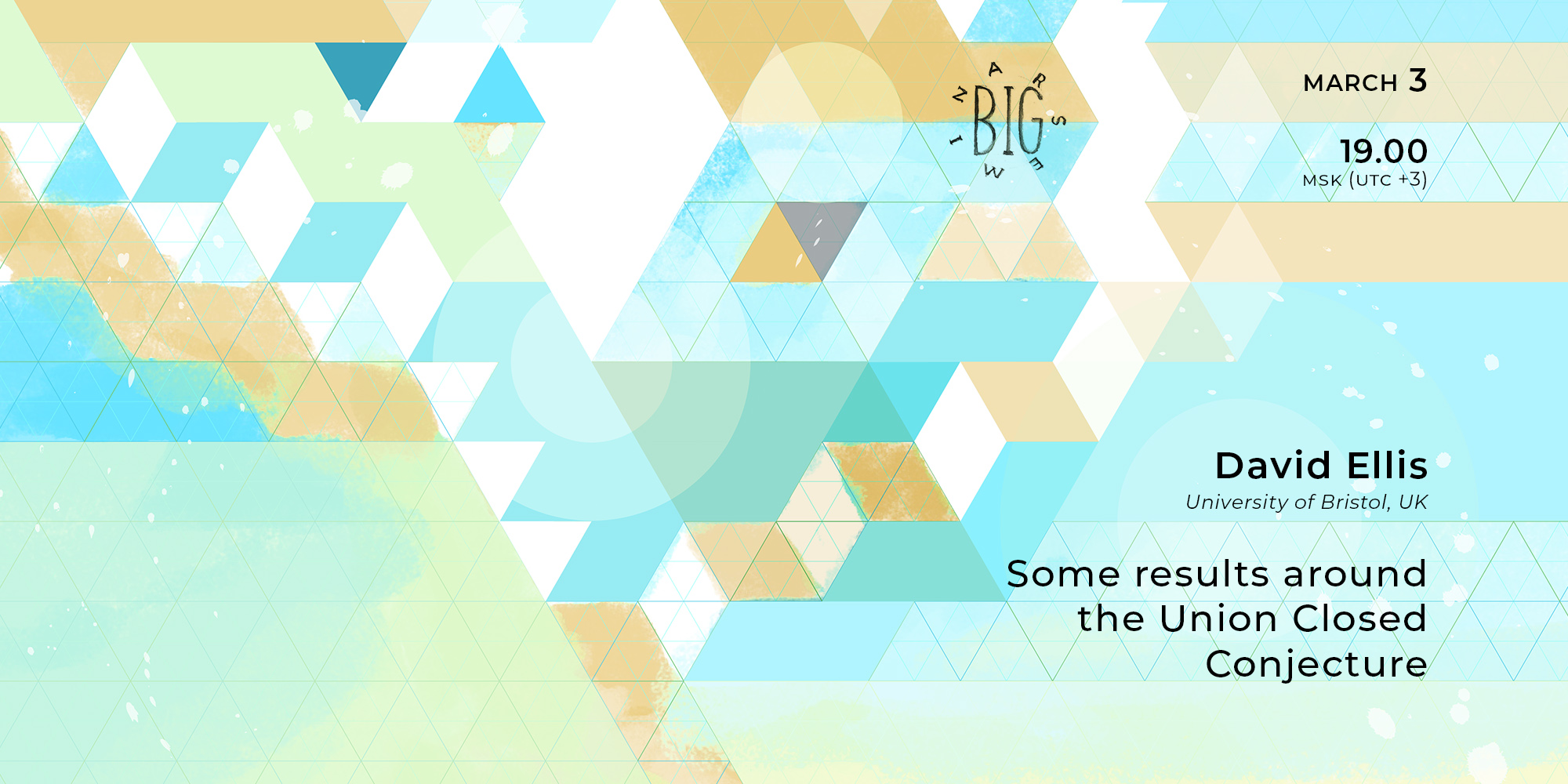# David Ellis “Some results around the Union Closed Conjecture” | Big Seminar# David Ellis "Some results around the Union Closed Conjecture"

For unforeseen personal reasons, the speaker has had to postpone or cancel the seminar
The talk will be held in zoom
Password: first 6 decimal places of $\pi$ after the decimal point
The celebrated Union Closed Conjecture of Peter Frankl states that if $F$ is a (non-trivial) union-closed family of subsets of a finite set $X$, then there exists an element of $X$ contained in at least half of the sets in $F$. Despite the efforts of many researchers over the last fifty years, and a recent Polymath project aimed at resolving it, the conjecture remains wide open. We discuss some recent results which demonstrate the limits of certain natural approaches to proving the conjecture (or to improving the known bounds for it). For example, we can show that for any positive integer $k$, there exists a finite union-closed family in which the (unique) smallest set has size $k$, but where each element of this set is contained in at most a $(((1+o(1))log_2(k))/(2k))$-fraction of all the sets in the family. This is asymptotically sharp, by results of Balla and of Wójcik. We will also discuss (if time permits) a strong counterexample to an approach (using 'average overlap densities') suggested in the Polymath project, and a newish (simple) proof of a very special case of the Union Closed Conjecture, viz., for union-closed families generated by all cyclic translates of a fixed subset of $Z/(nZ)$.